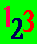The ABC Study Guide, University education in plain English alphabetically indexed. Click here to go to the main index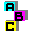## Measurement, Decimals and the Metric System

Measure
To measure something is to calculate how large it is, or how much of it there is, by comparing it with a fixed unit or with an object of a fixed size.

The Decimal System
The decimal system is the most widely used system of calculation and measurement. Decimus is Latin for tenth, and the decimal system is based on the number ten.

The Metric System
Metric is a French word related to the French word for
measure.

The metric system is a decimal measuring system that was made law in France during the French Revolution (adopted 1795) and is now used throughout the world.

The metric system uses the metre (a unit of length) as its basic unit. The litre is its basic unit of capacity, and the gram its basic unit of weight or mass.

Metre
My leg is about a metre long, but the inventors of the metric system wanted something far more reliable than legs and arms as the
fixed unit of their measurement. They chose the surface of our planet Earth.

A metre was defined as one ten-millionth of an imaginary line drawn from the north pole (through Paris) to the equator. This length was calculated, and a platinum bar of that length kept in Paris as the practical standard for legal purposes.

1 millimetre is 0.1 of a centimetre
1 centimetre is 0.1 of a decimetre
1 decimetre is 0.1 of a metre
1 metre is the basic unit of measure
1 decametre is 10 metres
1 hectometre is 10 decametres
1 kilometre is 10 hectometres

Litre
A litre is the basic unit for measuring volumes in the metric system. It is defined as a cube with sides that are one-tenth of
a metre long.

1 millilitre is 0.1 of a centilitre
1 centilitre is 0.1 of a decilitre
1 decilitre is 0.1 of a litre
1 litre is the basic unit of volume
1 decalitre is 10 litres
1 hectolitre is 10 decalitres
1 kilolitre is 10 hectolitres

Gram or Gramme
A gram (French for small weight) is the basic unit for weighing things in the metric system. It is defined as the weight of a cube of distilled water (at 4 degrees centigrade) with sides that are one-hundredth of
a metre long.

1 milligram is 0.1 of a centigram
1 centigram is 0.1 of a decigram
1 decigram is 0.1 of a gram
1 gram is the basic unit of weight
1 decagram is 10 grams
1 hectogram is 10 decagrams
1 kilogram is 10 hectograms

Hertz is the unit of frequency. The symbol for Herz is Hz. One hertz is one cycle a second. The light beam behind your computer screen must pass over the whole screen at least sixty times a second if the screen is not to flicker. The is described as a refresh rate of 60 Hz. The speed of computer processors is measured in megahertz. A megahertz is one million cycles per second. The symbol for megahertz is MHz.

The size of computer files and the storage capacity of a computer disk is measured in bytes. One byte is enough space to store one letter or character. If the naming conventions for the metric system were followed, a kilobyte would be one thousand bytes, just as a kilometre is a thousand meters and a kilogram is a thousand grams. Roughly, this is true. However, because the basic working of a computer is in twos (the binary system) instead of tens (the metric system) many people thought it would be good to have byte measurements in convenient numbers from the binary system. They make a kilobyte one thousand and 24 bytes because that is two multiplied by itself ten times (two to the power of ten). Both systems are in use.

1 kilobyte is one thousand bytes, or 1,024 bytes
1 megabyte is one million bytes, or 1,048,576 bytes
1 gigabyte is one thousand million bytes, or 1,073,741,824 bytes

How hot or cold something is is measured in relation to two points: The point at which a substance changes from a solid to liquid, or liquid to solid, state - freezing point - and the point at which a substance changes from a liquid to a vapour, or vapour to liquid, state - boiling point. Different substances change at different heats, so a common substance is used as the base: water.

The celsius (previously called centigrade) scale is a decimal scale. It calls the freezing point of pure water 0 degrees and the boiling point 100 degrees

The Réaumur scale is no longer used, but was once very popular in France and Germany. This calls boiling point 80 degrees

Engels, writing in 1845 for Germans, explained that social laws could be as certain as natural laws by arguing that

"If the influences demoralising to the working-man act more powerfully, more concentratedly than usual, he becomes an offender as certainly as water abandons the fluid for the vaporous state at 80 degrees, Réaumur."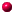Study links outside this site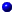Andrew Roberts' web Study GuidePicture introduction to this siteTop of Page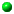Take a Break - Read a Poem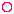Click coloured words to go where you want

Andrew Roberts likes to hear from users:
To contact him, please use the Communication Form

Maths index

*******************

cent = hundredth
centigram
centilitre
centimetre

deca = ten times
decagram
decalitre
decametre

deci = tenth
decigram
decilitre
decimetre

giga (giant)
= 1,000 million
Gigabyte

hecto = 100 times
hectogram
hectolitre
hectometre

kilo = 1,000 times
kilobyte
kilogram
kilolitre
kilometre

mega (great)
= million
Megabyte
Megahertz

metric = using ten
Metric

milli = thousandth
milligram
millilitre
millimetre

